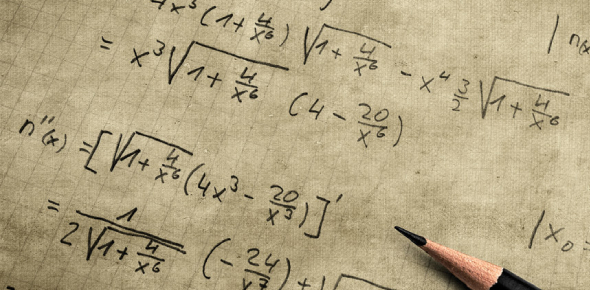# Calculus Math Exam Quiz!

9 Questions | Total Attempts: 78SettingsAre you adept when it comes to calculus? This math exam will put the spotlight on one of the most challenging subjects in mathematics. Calculus is the study of constant change. It deals with acquiring properties of derivatives and integrals of functions. There are two significant types of calculus. These include differential calculus and integral calculus. This quiz will demonstrate your knowledge of calculus and see whether your numbers add up.

• 1.
Determine: lim(x->4)[(x2-16)/(x-4)]  [Blank]
• 2.
Use first principles to determine the derivative of f if:
1. f(x)=x2+5 [Blank]
2. f(x)=-2x2  [Blank]
3. f(x)=x2/5 [Blank]
• 3.
Use the rules of derivatives to determine the derivative of g if:
1. g(x)=x2+3x+5 [Blank]
2. g(x)=5x2-x-3  [Blank]
• 4.
The graphs of the parabola f(x)=3x2+2, and the straight lines g and h are represented in the sketch. g is a tangent to f at the point A and h is perpendicular to g at A. The coordinates of A are (1;5).
1. Determine the equation of the tangent g
2. Determine the equation of h
round off at the final answer to 2 decimal places and use a full stop (.) as your decimal point g=[Blank] h=[Blank]
• 5.
A function f is defined by y=px3+5x2-qx-3.The graph has a turning point at (-2;9). Calculate the values of p and q
1. p= [Blank]
2. q=[Blank]
• 6.
The sketch alongside shows the curve of f(x)=x3-x2-8x+12 The curve has a y-intercept at (0;12) and turning points at (2;0) and B. The point A is an x-intercept SEPARATE COORDINATES WITH ;
1. Calculate the Coordinates of A
2. Calculate the x-coordinate of B
3. Calculate the coordinates of the point of inflection
A= [Blank] x coordinate of B=[Blank] POF=[Blank]
• 7.
A factory has x employees and makes a profit of P rand per week. The relation between the profit and the number of employees is expressed by the formula P=-2x3+600x+1000 Calculate:
1. The number of employees, x, for the factory to make a maximum profit
2. The maximum profit
x=[Blank] P=[Blank]
• 8.
The height to which a plant grows during the first six months is given by the following function: f(x)=36x-3x2 ; 0<=x<=6 where x is the age of the plant in months and f(x) the height in centimeters above the ground after x months.
1. What height would the plant reach after 3 months? [Blank]
2. At the end of how many months will the plant reach its maximum height [Blank]
3. Hence calculate the maximum height to which the plant will grow [Blank]
• 9.
A function is defined by the equation y=x3+ax2+bx+c Q(0;4) is a turning point on the graph of y. And a local minimum occurs at a point R where y=0. P is a point on the graph with the coordinates (-1,0)
1. Calculate the values of a, b and c.
a= [Blank] b=[Blank] c=[Blank] and Determine the coordinates of R R=[Blank]
Related TopicsBack to top